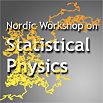#1st Nordic Workshop on Statistical Physics: Biological, Complex and Non-equilibrium Systems

Mar 17 – 19, 2010
Nordita
Europe/Stockholm timezone

## A Stochastic Model of Anomalous Heat Transport

Mar 17, 2010, 12:15 PM
45m
Nordita Seminar Room 132:028 (Nordita)

### Speaker

Carlos Mejia-Monasterio

### Description

Abstract: We investigate the non-equilibrium dynamics of a chain of harmonic oscillators in contact with two stochastic Langevin heat baths at different temperatures and undergoing random collisions between neighbours that exchange their momenta with a constant rate $\gamma$. By means of an appropriate continuum limit, we solve the equations for the covariance matrix to leading order in the stationary state, and derive exact expressions for the temperature profile and for the leading contribution of the energy current, which scales as $1/\sqrt{\gamma N}$. At finite times, we solve adiabatically the equation describing the time evolution of the temperature profile $T(y,t)$, obtaining that in the bulk of the system, $T(y)$ evolves according to the energy continuity equation, but with a space-time scaling that is described by a fractional diffusion equation.

### Presentation materials

There are no materials yet.